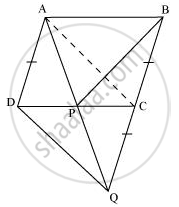# In the following figure, ABCD is parallelogram and BC is produced to a point Q such that AD = CQ. If AQ intersect DC at P, show that - Mathematics

In the following figure, ABCD is parallelogram and BC is produced to a point Q such that AD = CQ. If AQ intersect DC at P, show that

ar (BPC) = ar (DPQ).

[Hint: Join AC.]#### Solution

It is given that ABCD is a parallelogram.

AD || BC and AB || DC(Opposite sides of a parallelogram are parallel to each other)

Join point A to point C.Consider ΔAPC and ΔBPC

ΔAPC and ΔBPC are lying on the same base PC and between the same parallels PC and AB. Therefore,

Area (ΔAPC) = Area (ΔBPC) ... (1)

In quadrilateral ACDQ, it is given that

Since ABCD is a parallelogram,

AD || BC (Opposite sides of a parallelogram are parallel)

CQ is a line segment which is obtained when line segment BC is produced.

We have,

AC = DQ and AC || DQ

Hence, ACQD is a parallelogram.

Consider ΔDCQ and ΔACQ

These are on the same base CQ and between the same parallels CQ and AD. Therefore,

Area (ΔDCQ) = Area (ΔACQ)

⇒ Area (ΔDCQ) − Area (ΔPQC) = Area (ΔACQ) − Area (ΔPQC)

⇒ Area (ΔDPQ) = Area (ΔAPC) ... (2)

From equations (1) and (2), we obtain

Area (ΔBPC) = Area (ΔDPQ)

Concept: Theorem: Parallelograms on the Same Base and Between the Same Parallels.
Is there an error in this question or solution?
Chapter 9: Areas of Parallelograms and Triangles - Exercise 9.4 [Page 165]

#### APPEARS IN

NCERT Class 9 Maths
Chapter 9 Areas of Parallelograms and Triangles
Exercise 9.4 | Q 4 | Page 165

Share